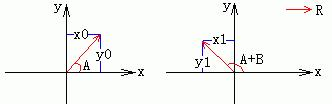• 二维坐标系下的向量旋转：  在2-D的迪卡尔坐标系中，一个位置向量的旋转公式可以由三角函数的几何意义推出。比如上图所示是位置向量R逆时针旋转角度B前后的情况。 在左图中，我们有关系： x0 = |R| * cosA...
二维坐标系下的向量旋转：(a)                                                     (b)
在二维坐标系中，向量的旋转公式可以由三角函数的几何意义推出。
比如上图所示是位置向量R逆时针旋转角度B前后的情况。
在(a)图中，假设向量的模长为R, 则可以推导出如下关系：
x0 = R  * cosA
y0 = R  * sinA
变换之后：
cosA = x0  /  R
sinA = y0  /  R
在(b)图中， 同理可以可证：
x1 = R * cos（ A + B ）
y1 = R * sin（ A + B ）
其中向量（x1， y1）为向量（x0， y0）逆时针旋转角度B后得到的点。
然后根据三角函数的表达式， 将cos（A+B）和sin（A+B）分解，得到
x1 = R  * （ cosAcosB - sinAsinB ）
y1 = R * （ sinAcosB + cosAsinB ）
现在把
cosA = x0  /  R
sinA = y0 /  R
代入上面的式子，得到
x1 = R * （x0 * cosB  /  R - y0 * sinB  /  R）
y1 = R * （y0  * cosB  /  R + x0 * sinB  /  R）
整理：
x1 = x0 * cosB - y0 * sinB
y1 = x0 * sinB + y0 * cosB
这样我们就得到了向量围绕坐标原点的逆时针旋转公式。

同理可知：
顺时针旋转公式如下：
x1 = x0 * cos（-B） - y0 * sin（-B）
y1 = x0 * sin（-B） + y0 * cos（-B）
整理：
x1 = x0 * cosB + y0 * sinB
y1 = -x0 * sinB + y0 * cosB


展开全文• score """ 导入数据 """ df = pd.read_csv('C:\\Users\\asus\\Desktop\\数据\\zhiyintu.csv') X2=df.iloc[:,4:6] #比值系数 X3=df.iloc[:,8:11] #单位向量 #X4=df.iloc[:,6:8] #比值系数 X5 = np.array(X2) y1=df['...
import numpy as np
import pandas as pd
import matplotlib.pyplot as plt
from  matplotlib.colors import ListedColormap
from sklearn.preprocessing import StandardScaler
from sklearn.datasets import make_moons,make_circles,make_classification
from sklearn.neighbors import KNeighborsClassifier
from sklearn.svm import SVC
from sklearn.tree import DecisionTreeClassifier
from sklearn.naive_bayes import GaussianNB
from sklearn.discriminant_analysis import LinearDiscriminantAnalysis as LDA
from sklearn.discriminant_analysis import QuadraticDiscriminantAnalysis as QDA
from sklearn import svm
import matplotlib as mpl
from sklearn import tree
from sklearn import neighbors
from sklearn.metrics import confusion_matrix
from sklearn.ensemble import BaggingClassifier
from sklearn.preprocessing import label_binarize
from sklearn.multiclass import OneVsRestClassifier
from sklearn.model_selection import train_test_split,cross_val_score
from sklearn.metrics import accuracy_score,roc_curve,auc
from sklearn.ensemble import VotingClassifier
from sklearn.metrics import accuracy_score
"""
导入数据
"""
X2=df.iloc[:,4:6]   #比值系数
X3=df.iloc[:,8:11]  #单位向量
#X4=df.iloc[:,6:8]   #比值系数
X5 = np.array(X2)
y1=df['guzhang']
"""
构造训练数据
"""
XD=[]
yD=[]
datasets =[]
for i in range(len(y1)):
XX=X3[i:i+1]
yy=y1[i:i+1]
XXX = np.tile(np.array(XX),(30,1)) + abs(np.tile(np.array(XX),(30,1)))*0.2*np.random.randn(1*30,3)
yyy = np.tile(np.array(yy),(30))
XD.append(XXX)
yD.append(yyy)
data=(XXX,yyy)
datasets.append(data)
#下面代码将每一类的故障数据集合到一起
XXXX=np.r_[XD,XD,XD,XD,XD,XD,XD,XD,XD,XD,XD,XD,XD]
yyyy=np.r_[yD,yD,yD,yD,yD,yD,yD,yD,yD,yD,yD,yD,yD]

from matplotlib import pyplot as plt
from mpl_toolkits.mplot3d import Axes3D
fig = plt.figure(figsize=(20,14))  #定义新的三维坐标轴
ax = plt.axes(projection='3d')

ax.set_zlabel('Z', fontdict={'size': 15, 'color': 'B'})
ax.set_ylabel('Y', fontdict={'size': 15, 'color': 'B'})
ax.set_xlabel('X', fontdict={'size': 15, 'color': 'B'})

'#800080','#556B2F','#008000','#FF0000','#32CD32','#48D1CC']
import matplotlib.font_manager as fm
myfont=fm.FontProperties(fname='C:/Windows/Fonts/msyh.ttc')
for i,k,j in zip(datasets,colvalue,range(13)):
X,y=i
ax.scatter(X[:,0],X[:,1],X[:,2],c=k,label="故障{}".format(j+1) )    #生成散点.利用c控制颜色序列,s控制大小
ax.legend(prop=myfont,loc='best')
plt.show()


展开全文• C# WPF动点任意移动气泡画法(解决方案使用到数学勾股定理、正弦定理、向量知识)。
C# WPF动点任意移动气泡画法(解决方案使用到数学勾股定理、正弦定理、向量知识)。

参考文章：
（1）C# WPF动点任意移动气泡画法(解决方案使用到数学勾股定理、正弦定理、向量知识)。
（2）https://www.cnblogs.com/BarryJames/p/7885564.html
备忘一下。


展开全文• C# WPF动点任意移动气泡画法(解决方案使用到数学勾股定理、正弦定理、向量知识)。 原文:C# WPF动点任意移动气泡画法(解决方案使用到数学勾股定理、正弦定理、向量知识)。 许久没写博客了，最近在研究...


C# WPF动点任意移动气泡画法(解决方案使用到数学勾股定理、正弦定理、向量知识)。

原文:
C# WPF动点任意移动气泡画法(解决方案使用到数学勾股定理、正弦定理、向量知识)。

许久没写博客了，最近在研究WPF下气泡的画法，研发过程还是比较艰辛的（主要是复习了高中的数学知识，MMP全忘光了），这篇博客主要是提供一个思路给大家参考，如果有大神还有更好的解决方案可以不吝您的言论尽情留言。拿个这个类型的功能项目，首先分析可以假设气泡是由：椭圆/矩形/圆(椭圆的特例)和三角形组成，OK首先分步骤介绍研发步骤：
第一：首先我的所有的图形都是基于矩阵画出来的，坐标轴起点是(0,0),假设一个拖拉点DynamicPoint (x,y),和一个固定点FixedPoint (m,n);由两点即可确定一个矩形大小，从里面画出内接图形和一个三角形；
1、新建矩形  var TriagleRect = new Rect(FixedPoint, DynamicPoint);
2、假设矩形之内存在一个等比例大小的圆，而圆是由圆心和半径组成的直线所划过的弧确定的，半径R，圆心CenterXY (p,q);相当于已知
3、可以移动的点P设为：CurrentFixedPoint(s,t); 这个点是由鼠标捕获的相当于已知;
4、由动点CurrentFixedPoint(s,t)向圆 M（圆心为CenterXY (p,q),半径R）作两条圆的切线，求出两切点F1(f1x,f1y)、F2(f2x,f2y)坐标值?
如下图(做的图比较难看，做辅助之用)到这边肯定很多人觉得很熟悉，没错这是高中的数学题，这边在研究的过程中研究了两种解决方案，下面简单的介绍下：
方案1：
如图，由圆心点C向两切点做垂直线，然后根据三角函数做辅助线PQ，QC得出斜边PC长，由图中可以知道
double Sine = R / AB; //求出正弦值
∠F1PC= Math.Round((Math.Asin(Sine) / Math.PI) * 180, 2);//把正弦值换算成角度
利用向量和向量模进行计算二元一次方程可以得出F1,F2坐标.
方程1：PF1向量=PC向量+CF1向量  ，这里可以得出一个关于F1的二元一次方程；
方程2：PF1向量的模=（PC平方+CF1平方）开根号,这里可以得出一个关于F1的二元二次方程；
由这两个方程式可以解出F1的坐标值，同理也可以得出F2的坐标值;
方案2：
也是如图，方案1是稍微复杂了一点，比较不利于软件当中的应用，这边着重介绍第二种算出切点的可行性方案；
double MB = Math.Sqrt(Math.Pow(PC, 2) - Math.Pow(R, 2));//MB为切线的长度
double Sine = R / AB; //求出正弦值
∠F1PC= Math.Round((Math.Asin(Sine) / Math.PI) * 180, 2);//把正弦值换算成角度
接下来跟方案1不同的地方是：我要让圆心点按照角度∠F1PC进行顺逆时针进行旋转

1  //把移动点作为圆心按照角度SineAngle旋转，有方向，顺逆时针
2             Vector vector = Point.Subtract(CenterXY, CurrentFixedPoint);
3             Matrix matrix = new Matrix();
4             matrix.RotateAt(SineAngle, CurrentFixedPoint.X, CurrentFixedPoint.Y);
5             //转换成单位向量1
6             var v = matrix.Transform(vector);
7             v.Normalize();
8
9             Matrix matrix2 = new Matrix();
10             matrix2.ScaleAt(_vector, _vector, CurrentFixedPoint.X, CurrentFixedPoint.Y);
11             var v2 = matrix2.Transform(v);
12             return v2;

经过上面旋转，然后缩放到单位1的向量假设为PF1' ,然后按比例放大到PF1的模长之后得出向量PF1，这样就可以得出F1点坐标，同理得出F2；具体转换如下：

1  //根据获得的向量值求出切点的坐标值
2             var tmpPoint = Point.Add(P, SecondPoint);
3             var tmpPoint2 = Point.Add(P, ThirdPoint);

这样就实现了移动动点P，F1,F2也会跟着角度进行实时变化，但是夹角不变，OK，现在动态的两个点都已经确认出来了，接下来就可以通过C#提供的方法进行连线，这样就组成了一个三角形，这个三角形是由内圆心出发延伸至动点P的图形。

1 //开始连线画点
2             PathFigure PointPathFigure = new PathFigure();
3             PointPathFigure.StartPoint = CurrentFixedPoint;
4             PointPathFigure.Segments.Add(new LineSegment(new Point(tmpPoint.X, tmpPoint.Y), true));
5             PointPathFigure.Segments.Add(new LineSegment(new Point(tmpPoint2.X, tmpPoint2.Y), true));
6
7             PathGeometry myPathGeometry = new PathGeometry();

第二：两个独立图形出来之后那就是组合图形了，C#组合图形提供了一个专门的方法：Geometry.Combine(Geometry geometry1, Geometry geometry2, GeometryCombineMode mode, Transform transform)，不多说不懂得可以查阅资料; 备注：ellipse 是第一个形状，四个中心点分别位于矩阵的边上；

1             //组合图形
2             var geometry = Geometry.Combine(myPathGeometry, ellipse, GeometryCombineMode.Union, null);
3
4             this.DrawGeometry(streamGeometryContext, geometry);

效果图如下：下面是任意移动动点P之后的效果。个人思路仅供参考。（这是原创文章，如有转载，请备注清楚）posted on
2017-11-24 10:17
NET未来之路 阅读(
...) 评论(
...)
编辑
收藏

转载于:https://www.cnblogs.com/lonelyxmas/p/7889271.html
展开全文•canvas
• 编译环境: ubuntu 14.04, qtCreator 4.8, pcl 1.8, opencv2.4.9 input: 双边滤波处理后的深度图（图像大小640*480,深度值范围0~2.226）生成的txt文件（＂DEPTH_METRIC_...output: 法向量可视化二位图和三维点云数据
• 听上去很简单，但是这条线我们可以用不一样的画法画，哪条线才是最合适？ 除了界限，样本与线的距离同样有意义，它代表着样本分类的可信程度，以苹果这一侧为例，与线距离最远的苹果是苹果的可能性最高；离得越近是...
• ## MATLAB绘制向量图

千次阅读 2019-08-03 09:41:37
MATLAB绘制向量图 对于如何在二维平面中绘制带箭头的向量，先给出一个实例： clear; clc; A=[4,5]; B=[-10,0]; C=A+B; M=[A;B;C]; X=[M(:,1);A(1)]; Y=[M(:,2);A(2)]; plot(X,Y,'r'); axis ...
• 支持向量机 有监督学习 分类问题 VapnikVapnikVapnik 线性可分支持向量机/线性支持向量机/非线性支持向量机 什么是支持向量机呢？ Support Vector MachineSupport\space Vector\space MachineSupport&...svm 统计学习方法 sklearn
• 在画线段时，应该把线段理解成向量，因为线段也是有大小，有方向。 并且建立一个线段的类，因为每条线段都是不同的对象。线段类的x,y属性就是线段的起点，再添加两个属性，记录线段的终点。下面是一个简单的例子，...Flash
• 下面展示一种在三维点云上可视化法向量的方法，将单位向量映射到RGB立方体上相应的RGB颜色。用于将三维点云或网格数据上的法向矢量可视化为彩色，而不是法向量箭头因为有时很难看清楚箭头的指向。 对于点云中的一个...可视化 3D点云
• Class SVM}') xlabel('Sepal Length (cm)') ylabel('Sepal Width (cm)') legend('Observation','Support Vector') hold off 分析 其实, 散点图很容易画, 大家都各有各的画法.主要问题是分界线的绘制, 因为得到分类器...机器学习 模式识别
• 用斜二测画法画直观图，本质上就是把三维空间中图形的每个点投影到一个二维...当年学向量的时候推导了一些变换公式（当时还不知道矩阵乘法），顺便研究了一下怎么样通过计算画直观图而不是凭感觉。乱搞了一通蒙出一...
• 什么是有穷状态向量机 2.有穷状态向量机的定义 具体的含义： 画法 例题： 二。正则文法： 右线性文法与状态图的转换： 文法转状态图：先画出所有的非终结符，然后再人为添加一个结束集合，然后再添加起始...
• 向量可视化 法向量可视化 概念 显示任意物体的法向量。当编写光照着色器时，你可能会最终会得到一些奇怪的视觉输出，但又很难确定导致问题的原因。光照错误很常见的原因就是法向量错误，这可能是由于不正确加载...着色器
• 三分钟理解零序电流保护向量图 --ujslili 1.正向故障 疑问？ 2.反方向故障： 3.接线方式 4.零序电流保护的评价 优点： 1）灵敏度高——几乎不受负荷电流影响——影响零序电流的就只有变压器阻抗和线路 阻抗...
• 风螺旋线的画法比较（三） 2016-01-23 刘崇军 风螺旋线 　风螺旋线的画法中有一种经常出现但并未完全覆盖风螺旋范围的画法，我称之为直线段模拟画法。 　该画法的绘制过程是这样的： 　从圆心出发，每隔一定...
• 前言 word2vec是如何得到词向量的？这个问题比较大。...得到你想要的processed corpus之后，将他们的one-hot向量作为word2vec的输入，通过word2vec训练低维词向量（word embedding）就ok了。不得不说word2ve
• 2、求出延迟禁止表和冲突向量； 3、构造流水线状态转移图； 4、寻找最小等间隔调度； 5、寻找最佳调度，并给出最小平均延迟和流水线的最大吞吐率； 按此流水线调度方案，输入n个任务，画出时空图，求实际吞吐率（选...
• 判断两向量的夹角方向，用向量叉乘来判断 假设有两向量(v1x,v1y), (v2x, v2y) 坐标系是右手坐标系，0点在屏幕左下方，往右是x正方向，往上是y正方向 角度逆时针为正，顺时针为负 从v1 到 v2的夹角是顺时针还是逆时针...
• 计算出点（x1,y1）和点（x3,y3）的中心作为新坐标的原点，这两点的连线作为x轴，其法向量取为y轴。
• X2=df.iloc[:,4:6] #比值系数 #X2=df.iloc[:,6:8] #比值系数 X3=df.iloc[:,8:11] #单位向量 y1=df['guzhang'] XD=[] yD=[] datasets =[] for i in range(len(y1)): XX=X2[i:i+1] yy=y1[i:i+1] XXX = np.tile(np....
• 向量 lab在此 notebook 中，你将学习如何绘制二维向量和进行某些向量计算。具体来说：绘制二维向量将二维向量与标量相乘并绘制结果将两个向量相加并绘制结果对于此 lab，我们将使用 python 软件包 NumPy 创建向量并...
• 用上图来作为一个简单的例子，图中有三角和圆两个类别，我们想找一条线把他们分开，当然这条线有很多种画法，图中只列出简单的三种分法，此时我们可以看出用一条直线我们可以很好的将两种类别分开，此时我们就可以说...机器学习 SVM...